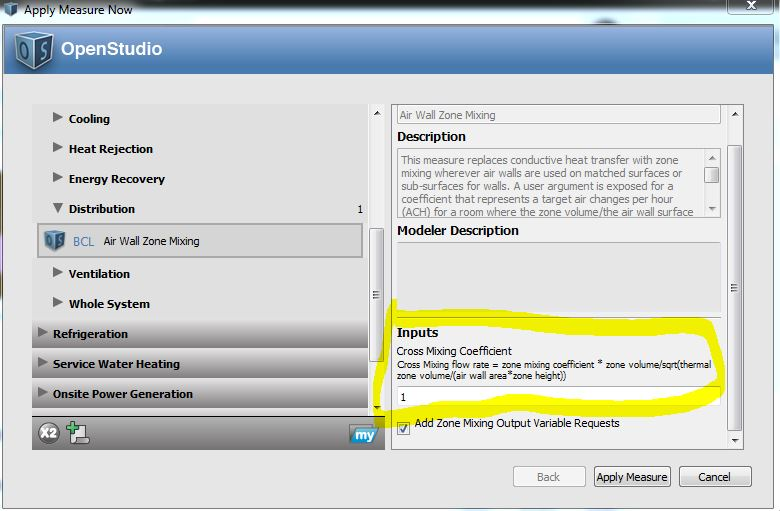Question-and-Answer Resource for the Building Energy Modeling Community
Get started with the Help page

apply measure air wall zone mixing

can someone help me with the meaning of these terms to calculate ''cross mixing coeffient. How can i calculate all this terms??edit retag close merge delete

Sort by » oldest newest most voted

@day9914, This measure was made when air mixing was added to OpenStudio to test air mixing and demonstrate an alternative approach to modeling zone boundaries in core and perimeter models where the zone boundary doesn't represent a physical wall. Traditionally there is just conductive heat transfer, but this measure instead changes the boundary condition to adiabatic and only uses air mixing to thermally connect the zones. This measure just applies a simple formula with a user adjustable coefficient (cross mixing coefficient) to apply a multiplier to the auto-calculated value. A number of factors that may not be evident from the model inputs will impact the actual realized air mixing in the building, and this measure does not attempt to determine a precise value.

The basic formula looks at the zone volume, area of the connecting surface, and the zone height. Using the default coefficient of 1, a space that is as high as it is deep (in direction away from the connecting wall) will have about 1 ACH of mixing. If you increase the depth by a factor of 4, the ACH will drop in half, although because of the increased volume there will still be twice as much CFM as with the original space. There could certainly be better formulas, but this was just something to start with.

I did run a prototype medium office in Houston, and then swept from 0 to 10 for an air mixing coefficient. Using a coefficient of 0 (no air or conductive transfer) resulted in a 1% decrease of energy consumption versus the prototype building, while a coefficient of 10 resulted in a 2% increase in consumption. Somewhere between 1 and 2.5 for the coefficient resulted in a similar EUI as the un-altered prototype building. But in all cases the peak load was higher with air mixing than in the baseline model. The lowest increase in peak load is with a coefficient of 0.5 to 1.0.

The measure is distributed with a PDF, but that had some inconsistencies and outdated values in it. I have a newer markdown file, which I have pasted below. This will eventually ship with the measure. As a note, while this measure focuses on cross mixing of equal values between zones, there is a different measure that deals with zone ventilation and air mixing in one direction from external zones to and then up through an atrium.

Measure Intent

• This measure is meant to provide a simple approximation of zone mixing where the zone boundary doesn’t represent a physical wall.
• Typically the “Air Wall” construction In OpenStudio is mainly used if using Radiance for daylighting. For thermal analysis it is modeled as something similar to plywood.
• This measure uses air walls to define where zone mixing should be used instead of conductive heat transfer.

Seed Model Preparation

• Create a model with air walls for zone boundaries that don’t represent physical walls.
• If you have a core and perimeter model you can just ...
more